Open In App
Related Articles

# Find the first non-repeating character from a stream of characters

Given a stream of characters, find the first non-repeating character from the stream. You need to tell the first non-repeating character in O(1) time at any moment.

If we follow the first approach discussed here, then we need to store the stream so that we can traverse it one more time to find the first non-repeating character at any moment. If we use the extended approach discussed in the same post, we need to go through the count array every time the first non-repeating element is queried. We can find the first non-repeating character from the stream at any moment without traversing any array.

The following problem can be solved using two methods:
Method 1: Using Hashmap to keep Track of the character already encountered:

The idea is to maintain a hashmap that uses constant space of at max 26 entries. This will keep the track of characters already encountered in the string and do so in constant query time. Secondly, an ArrayList or Vector can be used to keep track of the current unique characters from the beginning which should be added to the resultant string. Whenever any unique character is encountered again, it’s removed from the vector, but kept in HashMap to mark it as encountered. If the list is empty at any point, this means there is no non-repeating character present in the string, hence ‘#’ can be added.

Below is the implementation of the above code:

## C++

 `#include ``using` `namespace` `std;``string FirstNonRepeating(string A)``{``    ``vector<``char``> v;``    ``unordered_map<``char``, ``int``> mp;``    ``string ans;` `    ``for` `(``char` `ch : A) {``        ``if` `(mp.find(ch)``            ``== mp.end()) { ``// any new character visited for``                           ``// the first time``            ``v.push_back(ch);``            ``mp[ch] = 1;``        ``}``        ``else` `{``            ``// any repeated character visited``            ``int` `index``                ``= find(v.begin(), v.end(), ch) - v.begin();``            ``// for any repeated character encountered more``            ``// than twice the index will be equal to``            ``// v.size()``            ``if` `(index < v.size())``                ``v.erase(v.begin() + index);``        ``}``        ``ans += (v.empty() ? ``'#'` `: v.front());``    ``}` `    ``return` `ans;``}``int` `main()``{``    ``string A = ``"geeksforgeeksandgeeksquizfor"``;``    ``string ans = FirstNonRepeating(A);``    ``cout << ans << endl;``}`

## Java

 `// Java implementation for the above approach``import` `java.io.*;``import` `java.util.*;` `class` `GFG {` `    ``static` `String FirstNonRepeating(String A)``    ``{``          ``// Arraylist to keep track of current unique characters``          ``// Hashmap to keep track of character encountered at least once``          ``ArrayList list = ``new` `ArrayList<>();``        ``HashMap map = ``new` `HashMap<>();``        ``StringBuilder sb = ``new` `StringBuilder();` `        ``for` `(``char` `ch : A.toCharArray()) {``            ``if` `(!map.containsKey(ch)) { ``// any new character encountered first time``                ``list.add(ch);``                ``map.put(ch, ``1``);``            ``}``            ``else` `{``                  ``//any repeated character encountered``                ``int` `index = list.indexOf(ch);``              ` `                ``// for any repeated character encountered more than twice the``                  ``// index will be -1``                ``if` `(index != -``1``)``                      ``list.remove(index);``            ``}``            ``sb.append(list.isEmpty() ? ``'#'` `: list.get(``0``));``        ``}``        ``return` `sb.toString();``    ``}` `    ``public` `static` `void` `main(String[] args)``    ``{``        ``String A = ``"geeksforgeeksandgeeksquizfor"``;``        ``String ans = FirstNonRepeating(A);``        ``System.out.print(ans);``    ``}``}` `// This code is contributed by godcoder28.`

## Python3

 `def` `FirstNonRepeating(A):``        ``# Code here``        ``list` `=` `[]``        ``mp ``=` `{}``        ``ans ``=` `''` `        ``for` `ch ``in` `A:``            ``if` `ch ``not` `in` `mp:  ``# new character visited first time``                ``list``.append(ch)``                ``mp[ch] ``=` `1``            ``else``:``                ``# any repeated character encountered``                ``if` `ch ``in` `list``:``                    ``list``.remove(ch)``            ``ans ``+``=` `list``[``0``] ``if` `list` `else` `'#'` `        ``return` `ans``l ``=` `"geeksforgeeksandgeeksquizfor"``ans1 ``=` `FirstNonRepeating(l)``print``(ans1)`

## Javascript

 ``

## C#

 `using` `System;` `using` `System.Collections.Generic;` `public` `class` `GFG {``    ``static` `string` `FirstNonRepeating(``string` `A)``    ``{``        ``// List to keep track of current unique characters``        ``// Dictionary to keep track of character visited``        ``// at least once``        ``List<``char``> list = ``new` `List<``char``>();``        ``Dictionary<``char``, ``int``> map``            ``= ``new` `Dictionary<``char``, ``int``>();``        ``string` `result = ``""``;` `        ``foreach``(``char` `ch ``in` `A)``        ``{``            ``if` `(!map.ContainsKey(``                    ``ch)) ``// any new character visited``                         ``// first time``            ``{``                ``list.Add(ch);``                ``map.Add(ch, 1);``            ``}``            ``else` `{``                ``// any repeated character visited``                ``int` `index = list.IndexOf(ch);``                ``if` `(index != -1) {``                    ``list.RemoveAt(index);``                ``}``            ``}``            ``result += (list.Count == 0 ? ``'#'` `: list);``        ``}` `        ``return` `result;``    ``}` `    ``static` `void` `Main(``string``[] args)``    ``{``        ``string` `A = ``"geeksforgeeksandgeeksquizfor"``;``        ``string` `ans = FirstNonRepeating(A);``        ``Console.WriteLine(ans);``    ``}``}`

Output

`ggggggggkkksfffffffffffffora`

Time Complexity: O(26 * n)
Auxiliary Space: O(n)

Method 2: Using Double Ended Linklist

The idea is to use a DLL (Doubly Linked List) to efficiently get the first non-repeating character from a stream. The DLL contains all non-repeating characters in order, i.e., the head of DLL contains first non-repeating character, the second node contains the second non-repeating, and so on.

We also maintain two arrays: one array is to maintain characters that are already visited two or more times, we call it repeated[], the other array is an array of pointers to linked list nodes, we call it inDLL[]. The size of both arrays is equal to alphabet size which is typically 256.

1. Create an empty DLL. Also, create two arrays inDLL[] and repeated[] of size 256. In DLL is an array of pointers to DLL nodes. repeated[] is a boolean array, repeated[x] is true if x is repeated two or more times, otherwise false. inDLL[x] contains a pointer to a DLL node if character x is present in DLL, otherwise NULL.
2. Initialize all entries of inDLL[] as NULL and repeated[] as false.
3. To get the first non-repeating character, return character at the head of DLL.
4. Following are steps to process a new character ‘x’ in a stream.
• If repeated[x] is true, ignore this character (x is already repeated two or more times in the stream)
• If repeated[x] is false and inDLL[x] is NULL (x is seen the first time). Append x to DLL and store address of new DLL node in inDLL[x].
• If repeated[x] is false and inDLL[x] is not NULL (x is seen a second time). Get DLL node of x using inDLL[x] and remove the node. Also, mark inDLL[x] as NULL and repeated[x] as true.

Note that appending a new node to DLL is O(1) operation if we maintain a tail pointer. Removing a node from DLL is also O(1). So both operations, addition of new character and finding first non-repeating character take O(1) time.

Below image is a dry run of the above approach: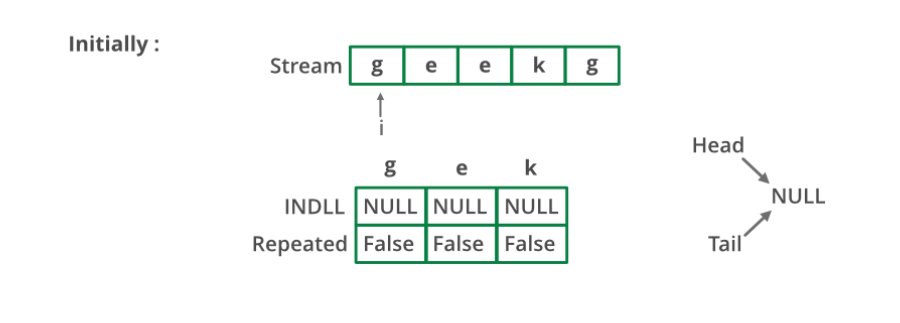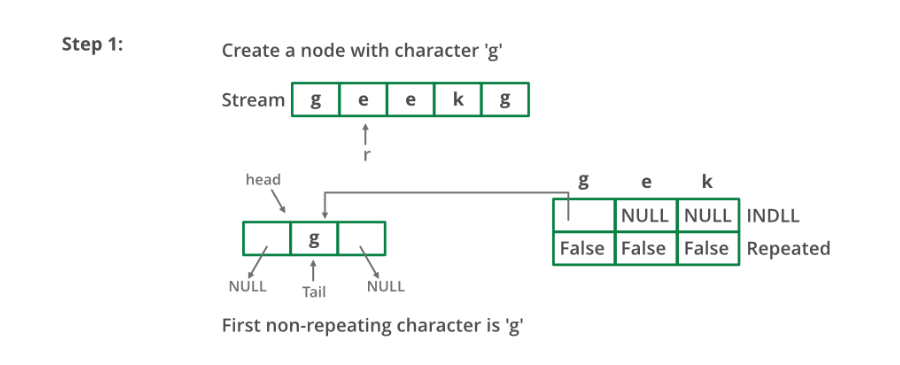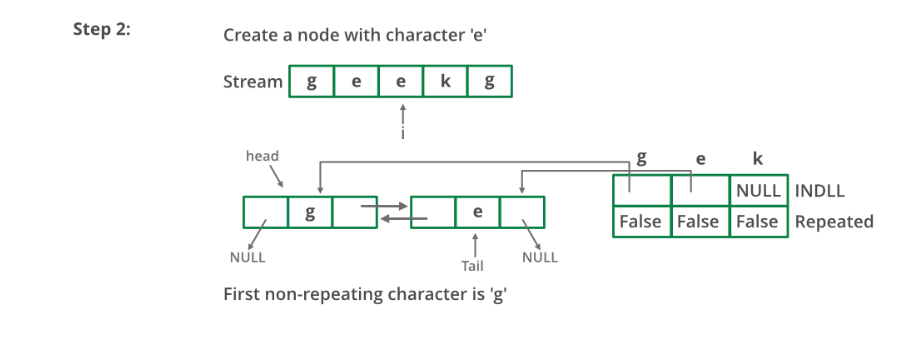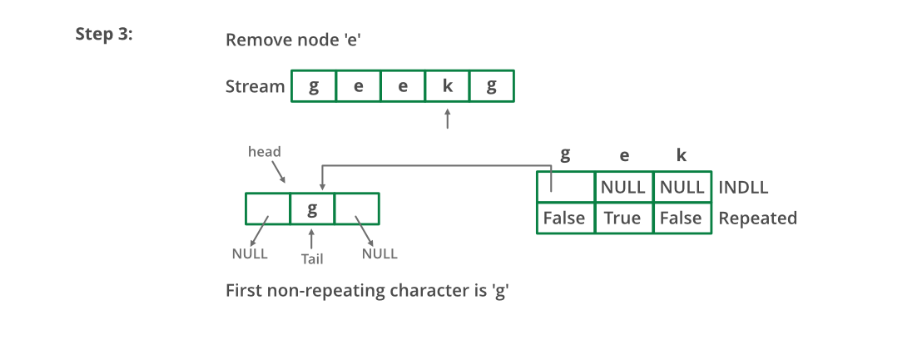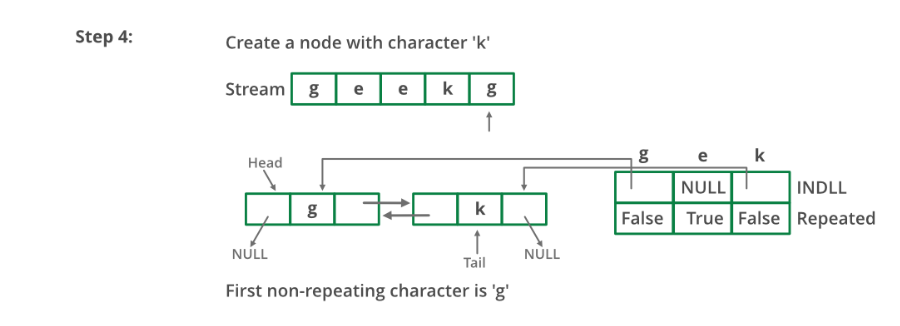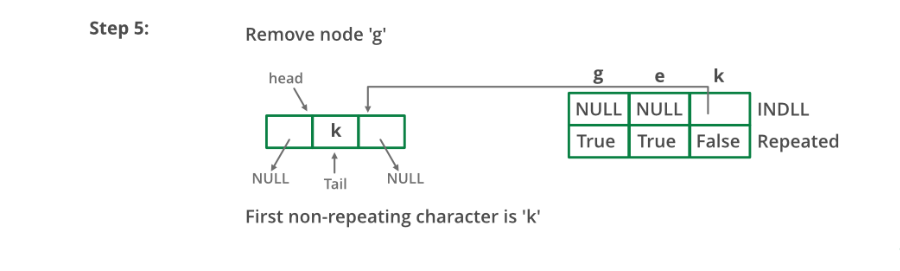Below is the implementation of the above approach:

## C++

 `// A C++ program to find first``// non-repeating character``// from a stream of characters``#include ``#define MAX_CHAR 256``using` `namespace` `std;` `// A linked list node``struct` `node {``    ``char` `a;``    ``struct` `node *next, *prev;``};` `// A utility function to append a character x at the end``// of DLL. Note that the function may change head and tail``// pointers, that is why pointers to these pointers are``// passed.``void` `appendNode(``struct` `node** head_ref,``                ``struct` `node** tail_ref, ``char` `x)``{``    ``struct` `node* temp = ``new` `node;``    ``temp->a = x;``    ``temp->prev = temp->next = NULL;` `    ``if` `(*head_ref == NULL) {``        ``*head_ref = *tail_ref = temp;``        ``return``;``    ``}``    ``(*tail_ref)->next = temp;``    ``temp->prev = *tail_ref;``    ``*tail_ref = temp;``}` `// A utility function to remove a node 'temp' from DLL.``// Note that the function may change the head and tail pointers,``// that is why pointers to these pointers are passed.``void` `removeNode(``struct` `node** head_ref,``                ``struct` `node** tail_ref, ``struct` `node* temp)``{``    ``if` `(*head_ref == NULL)``        ``return``;` `    ``if` `(*head_ref == temp)``        ``*head_ref = (*head_ref)->next;``    ``if` `(*tail_ref == temp)``        ``*tail_ref = (*tail_ref)->prev;``    ``if` `(temp->next != NULL)``        ``temp->next->prev = temp->prev;``    ``if` `(temp->prev != NULL)``        ``temp->prev->next = temp->next;` `    ``delete` `(temp);``}` `void` `findFirstNonRepeating()``{``    ``// inDLL[x] contains pointer to``    ``// a DLL node if x is present``    ``// in DLL. If x is not present, then inDLL[x] is NULL``    ``struct` `node* inDLL[MAX_CHAR];` `    ``// repeated[x] is true if x is repeated two or more``    ``// times. If x is not seen so far or x is seen only``    ``// once. then repeated[x] is false``    ``bool` `repeated[MAX_CHAR];` `    ``// Initialize the above two arrays``    ``struct` `node *head = NULL, *tail = NULL;``    ``for` `(``int` `i = 0; i < MAX_CHAR; i++) {``        ``inDLL[i] = NULL;``        ``repeated[i] = ``false``;``    ``}` `    ``// Let us consider following stream and see the process``    ``char` `stream[] = ``"geeksforgeeksandgeeksquizfor"``;``    ``for` `(``int` `i = 0; stream[i]; i++) {``        ``char` `x = stream[i];``        ``cout << ``"Reading "` `<< x << ``" from stream \n"``;` `        ``// We process this character only if it has not``        ``// occurred or occurred only once. repeated[x] is``        ``// true if x is repeated twice or more.s``        ``if` `(!repeated[x]) {``            ``// If the character is not in DLL, then add this``            ``// at the end of DLL.``            ``if` `(inDLL[x] == NULL) {``                ``appendNode(&head, &tail, stream[i]);``                ``inDLL[x] = tail;``            ``}``            ``else` `// Otherwise remove this character from DLL``            ``{``                ``removeNode(&head, &tail, inDLL[x]);``                ``inDLL[x] = NULL;``                ``repeated[x]``                    ``= ``true``; ``// Also mark it as repeated``            ``}``        ``}` `        ``// Print the current first non-repeating character``        ``// from stream``        ``if` `(head != NULL)``            ``cout << ``"First non-repeating character so far "``                    ``"is "``                 ``<< head->a << endl;``    ``}``}` `/* Driver code */``int` `main()``{``    ``findFirstNonRepeating();``    ``return` `0;``}`

## Java

 `// A Java program to find first non-repeating character``// from a stream of characters` `import` `java.util.ArrayList;``import` `java.util.List;` `public` `class` `NonReapeatingC {``    ``final` `static` `int` `MAX_CHAR = ``256``;` `    ``static` `void` `findFirstNonRepeating()``    ``{``        ``// inDLL[x] contains pointer to a DLL node if x is``        ``// present in DLL. If x is not present, then``        ``// inDLL[x] is NULL``        ``List inDLL = ``new` `ArrayList();` `        ``// repeated[x] is true if x is repeated two or more``        ``// times. If x is not seen so far or x is seen only``        ``// once. then repeated[x] is false``        ``boolean``[] repeated = ``new` `boolean``[MAX_CHAR];` `        ``// Let us consider following stream and see the``        ``// process``        ``String stream = ``"geeksforgeeksandgeeksquizfor"``;``        ``for` `(``int` `i = ``0``; i < stream.length(); i++) {``            ``char` `x = stream.charAt(i);``            ``System.out.println(``"Reading "` `+ x``                               ``+ ``" from stream \n"``);` `            ``// We process this character only if it has not``            ``// occurred or occurred only once. repeated[x]``            ``// is true if x is repeated twice or more.s``            ``if` `(!repeated[x]) {``                ``// If the character is not in DLL, then add``                ``// this at the end of DLL.``                ``if` `(!(inDLL.contains(x))) {``                    ``inDLL.add(x);``                ``}``                ``else` `// Otherwise remove this character from``                     ``// DLL``                ``{``                    ``inDLL.remove((Character)x);``                    ``repeated[x]``                        ``= ``true``; ``// Also mark it as repeated``                ``}``            ``}` `            ``// Print the current first non-repeating``            ``// character from stream``            ``if` `(inDLL.size() != ``0``) {``                ``System.out.print(``                    ``"First non-repeating character so far is "``);``                ``System.out.println(inDLL.get(``0``));``            ``}``        ``}``    ``}` `    ``/* Driver code */``    ``public` `static` `void` `main(String[] args)``    ``{``        ``findFirstNonRepeating();``    ``}``}``// This code is contributed by Sumit Ghosh`

## Python3

 `# A Python program to find first non-repeating character from``# a stream of characters``MAX_CHAR ``=` `256` `def` `findFirstNonRepeating():` `    ``# inDLL[x] contains pointer to a DLL node if x is present``    ``# in DLL. If x is not present, then inDLL[x] is NULL``    ``inDLL ``=` `[] ``*` `MAX_CHAR` `    ``# repeated[x] is true if x is repeated two or more times.``    ``# If x is not seen so far or x is seen only once. then``    ``# repeated[x] is false``    ``repeated ``=` `[``False``] ``*` `MAX_CHAR` `    ``# Let us consider following stream and see the process``    ``stream ``=` `"geekforgeekandgeeksandquizfor"``    ``for` `i ``in` `range``(``len``(stream)):``        ``x ``=` `stream[i]``        ``print` `(``"Reading "` `+` `x ``+` `" from stream"``)` `        ``# We process this character only if it has not occurred``        ``# or occurred only once. repeated[x] is true if x is``        ``# repeated twice or more.s``        ``if` `not` `repeated[``ord``(x)]:` `            ``# If the character is not in DLL, then add this``            ``# at the end of DLL``            ``if` `not` `x ``in` `inDLL:``                ``inDLL.append(x)``            ``else``:``                ``inDLL.remove(x)``                ``repeated[``ord``(x)] ``=` `True` `        ``if` `len``(inDLL) !``=` `0``:``            ``print` `(``"First non-repeating character so far is "``)``            ``print` `(``str``(inDLL[``0``]))` `# Driver program``findFirstNonRepeating()` `# This code is contributed by BHAVYA JAIN`

## Javascript

 ``

## C#

 `// A C# program to find first non-repeating character``// from a stream of characters``using` `System;``using` `System.Collections.Generic;` `public` `class` `NonReapeatingC {``    ``readonly` `static` `int` `MAX_CHAR = 256;` `    ``static` `void` `findFirstNonRepeating()``    ``{``        ``// inDLL[x] contains pointer to a DLL node if x is present``        ``// in DLL. If x is not present, then inDLL[x] is NULL``        ``List<``char``> inDLL = ``new` `List<``char``>();` `        ``// repeated[x] is true if x is repeated two or more times.``        ``// If x is not seen so far or x is seen only once. then``        ``// repeated[x] is false``        ``bool``[] repeated = ``new` `bool``[MAX_CHAR];` `        ``// Let us consider following stream and see the process``        ``String stream = ``"geeksforgeeksandgeeksquizfor"``;``        ``for` `(``int` `i = 0; i < stream.Length; i++) {``            ``char` `x = stream[i];``            ``Console.WriteLine(``"Reading "` `+ x + ``" from stream \n"``);` `            ``// We process this character only if it has not occurred``            ``// or occurred only once. repeated[x] is true if x is``            ``// repeated twice or more.s``            ``if` `(!repeated[x]) {``                ``// If the character is not in DLL, then add this at``                ``// the end of DLL.``                ``if` `(!(inDLL.Contains(x))) {``                    ``inDLL.Add(x);``                ``}``                ``else` `// Otherwise remove this character from DLL``                ``{``                    ``inDLL.Remove((``char``)x);``                    ``repeated[x] = ``true``; ``// Also mark it as repeated``                ``}``            ``}` `            ``// Print the current first non-repeating character from``            ``// stream``            ``if` `(inDLL.Count != 0) {``                ``Console.Write(``"First non-repeating character so far is "``);``                ``Console.WriteLine(inDLL);``            ``}``        ``}``    ``}` `    ``/* Driver code*/``    ``public` `static` `void` `Main(String[] args)``    ``{``        ``findFirstNonRepeating();``    ``}``}` `// This code has been contributed by 29AjayKumar`

Output

```...ng character so far is f
First non-repeating character so far is f
First non-repeating character so far is f
First non-repeating character so far is f
First non-repeating character so far is f
First non-repeating character so far is f
First non-repeating character so far is f
First non-repeating character so far is f
First non-repeating character so far is f
First non-repeating character so far is f
First non-repeating character so far is f
First non-repeating character so far is f
First non-repeating character so far is f
First non-repeating character so far is o
First non-repeating character so far is r
First non-repeating character so far is a```

Time Complexity: O(n)
Auxiliary Space: O(1)

Another approach :

This problem can be solved using queue, push into the queue every time when unique character is found and pop it out when you get front character of queue repeated in the stream , this is how first non-repeated character in managed.

Follow the below steps to solve the given problem:

• Take map to check the uniqueness of an element.
• Take queue to find first non-repeating element.
• Traverse through the string and increase the count of elements in map and push in to queue is count is 1.
• If count of front element of the queue > 1 anytime then pop it from the queue until we get unique element at the front.
• If queue is empty anytime append answer string with ‘#’ else append it with front element of queue.

Below is the implementation of the above approach:

## C++

 `#include ``using` `namespace` `std;` `string FirstNonRepeating(string A)``{` `    ``// ans string stores the final answer``    ``string ans = ``""``;``    ``// map to find uniqueness of an element``    ``unordered_map<``char``, ``int``> mp;``    ``queue<``char``> q;``    ``// queue to keep non-repeating element at the front.``    ``for` `(``int` `i = 0; i < A.length(); i++) {``        ``// if non-repeating element found push it in``        ``// queue and count in map``        ``if` `(mp.find(A[i]) == mp.end()) {``            ``q.push(A[i]);``        ``}``        ``mp[A[i]]++;``        ``// if anytime front element is repeating pop it``        ``// form queue``        ``while` `(!q.empty() && mp[q.front()] > 1) {``            ``q.pop();``        ``}``        ``// if queue is not empty append front element``        ``// else append "#" in ans string.``        ``if` `(!q.empty()) {``            ``ans += q.front();``        ``}``        ``else` `{``            ``ans += ``'#'``;``        ``}``    ``}``    ``// return ans``    ``return` `ans;``}` `int` `main()``{``    ``string A = ``"geeksforgeeksandgeeksquizfor"``;``    ``string ans = FirstNonRepeating(A);``    ``cout << ans << ``"\n"``;``    ``return` `0;``}`

## Java

 `// Java implementation for the above approach``import` `java.io.*;``import` `java.util.*;` `class` `GFG {` `    ``static` `String FirstNonRepeating(String A)``    ``{``        ``// ans string stores the final answer``        ``String ans = ``""``;``      ` `        ``// map to find uniqueness of an element``        ``Map mp = ``new` `HashMap<>();``        ``Queue q = ``new` `LinkedList<>();``      ` `        ``// queue to keep non-repeating element at the front.``        ``for` `(``int` `i = ``0``; i < A.length(); i++)``        ``{``          ` `            ``// if non-repeating element found push it in``            ``// queue and count in map``            ``if` `(!mp.containsKey(A.charAt(i))) {``                ``q.add(A.charAt(i));``            ``}``            ``mp.put(A.charAt(i),``                   ``mp.getOrDefault(A.charAt(i), ``0``) + ``1``);``          ` `            ``// if anytime front element is repeating pop it``            ``// form queue``            ``while` `(!q.isEmpty() && (mp.get(q.peek()) > ``1``)) {``                ``q.remove();``            ``}``          ` `            ``// if queue is not empty append front element``            ``// else append "#" in ans string.``            ``if` `(!q.isEmpty()) {``                ``ans += q.peek();``            ``}``            ``else` `{``                ``ans += ``'#'``;``            ``}``        ``}``        ``// return ans``        ``return` `ans;``    ``}` `    ``public` `static` `void` `main(String[] args)``    ``{``        ``String A = ``"geeksforgeeksandgeeksquizfor"``;``        ``String ans = FirstNonRepeating(A);``        ``System.out.print(ans);``    ``}``}` `// This code is contributed by lokeshmvs21.`

## Python

 `from` `collections ``import` `deque` `def` `FirstNonRepeating(A):``    ``ans ``=` `""``    ``mp ``=` `{}``    ``q ``=` `deque()``    ``for` `i ``in` `range``(``len``(A)):``        ``if` `A[i] ``not` `in` `mp:``            ``q.append(A[i])``        ``mp[A[i]] ``=` `mp.get(A[i], ``0``) ``+` `1``        ``while` `len``(q) > ``0` `and` `mp[q[``0``]] > ``1``:``            ``q.popleft()``        ``if` `len``(q) > ``0``:``            ``ans ``+``=` `q[``0``]``        ``else``:``            ``ans ``+``=` `"#"``    ``return` `ans` `A ``=` `"geeksforgeeksandgeeksquizfor"``ans ``=` `FirstNonRepeating(A)``print``(ans)`

## Javascript

 `function` `FirstNonRepeating(A)``{``    ``// ans let stores the final answer``    ``let ans = ``""``;``    ` `    ``// map to find uniqueness of an element``    ``let mp = ``new` `Map();``    ``for``(let i=97;i<123;i++)``    ``{``        ``mp.set(String.fromCharCode(i),0);``    ``}``    ``let q = [];``    ` `    ``// queue to keep non-repeating element at the front.``    ``for` `(let i = 0; i < A.length; i++)``    ``{``    ` `        ``// if non-repeating element found push it in``        ``// queue and count in map``        ``if` `(mp.get(A[i])==0) {``            ``q.push(A[i]);``        ``}``        ``mp.set(A[i],mp.get(A[i])+1);``        ` `        ``// if anytime front element is repeating pop it``        ``// form queue``        ``while` `(q.length>0 && mp.get(q)> 1) {``            ``q.shift();``        ``}``        ` `        ``// if queue is not empty append front element``        ``// else append "#" in ans string.``        ``if` `(q.length>0) {``            ``ans += q;``        ``}``        ``else` `{``            ``ans += ``'#'``;``        ``}``    ``}``    ` `    ``// return ans``    ``return` `ans;``}` `let A = ``"geeksforgeeksandgeeksquizfor"``;``let ans = FirstNonRepeating(A);``console.log(ans);` `// This code is contributed by akashish__`

## C#

 `using` `System;``using` `System.Collections.Generic;` `public` `class` `GFG {` `  ``public` `static` `string` `FirstNonRepeating(``string` `A)``  ``{` `    ``// ans string stores the final answer``    ``string` `ans = ``""``;` `    ``// map to find uniqueness of an element``    ``Dictionary<``char``, ``int``> mp``      ``= ``new` `Dictionary<``char``, ``int``>();``    ``Queue<``char``> q = ``new` `Queue<``char``>();` `    ``// queue to keep non-repeating element at the front.``    ``for` `(``int` `i = 0; i < A.Length; i++)``    ``{` `      ``// if non-repeating element found push it in``      ``// queue and count in map``      ``if` `(!mp.ContainsKey(A[i])) {``        ``q.Enqueue(A[i]);``      ``}``      ``if` `(mp.ContainsKey(A[i]))``        ``mp[A[i]] += 1;``      ``else``        ``mp[A[i]] = 1;` `      ``// if anytime front element is repeating pop it``      ``// form queue``      ``while` `(q.Count > 0 && mp[q.Peek()] > 1) {``        ``q.Dequeue();``      ``}` `      ``// if queue is not empty append front element``      ``// else append "#" in ans string.``      ``if` `(q.Count > 0) {``        ``ans += q.Peek();``      ``}``      ``else` `{``        ``ans += ``'#'``;``      ``}``    ``}` `    ``// return ans``    ``return` `ans;``  ``}` `  ``static` `public` `void` `Main()``  ``{` `    ``string` `A = ``"geeksforgeeksandgeeksquizfor"``;``    ``string` `ans = FirstNonRepeating(A);``    ``Console.WriteLine(ans);``  ``}``}` `// This code is contributed by akashish__`

Output

`ggggggggkkksfffffffffffffora`

Time Complexity: O(26 * n)
Auxiliary Space: O(n)

Using a Count Array:Follow the steps below to implement above idea:

• Create a count array of size 26 to store the frequency of each character.
• Create a queue to store the characters in the input stream.
• Initialize an empty string as the answer.
• For each character in the input stream, add it to the queue and increment its frequency in the count array.
• While the queue is not empty, check if the frequency of the front character in the queue is 1.
• If the frequency is 1, append the character to the answer. If the frequency is greater than 1, remove the front character from the queue.
• If there are no characters left in the queue, append ‘#’ to the answer.

Below is the implementation of above approach:

## C++

 `// C++ code to implement the above approach``#include ``#include ``#include ``using` `namespace` `std;``/*function to find first non-repeating character in a stream`` ``*/``string firstNonRepeatingChar(string input_stream)``{``    ``// Step 1: Create a count array of size 26 to store the``    ``// frequency of each character.``    ``int` `count = { 0 };``    ``// Step 2: Create a queue to store the characters in the``    ``// input stream.``    ``queue<``char``> q;``    ``// Step 3: Initialize an empty string as the answer.``    ``string answer = ``""``;` `    ``for` `(``char` `c :``         ``input_stream) { ``// Step 4: For each character in``                         ``// the input stream, add it to the``                         ``// queue and increment its``                         ``// frequency in the count array.``        ``count++; ``// Increment the frequency of the``                          ``// character in the count array``        ``q.push(c); ``// Add the character to the queue` `        ``while` `(!q.empty()``               ``&& count[q.front() - ``'a'``]``                      ``> 1) { ``// Step 5: While the queue is``                             ``// not empty, check if the``                             ``// frequency of the front``                             ``// character in the queue is 1.``            ``q.pop(); ``// Step 6: If the frequency is greater``                     ``// than 1, remove the front character``                     ``// from the queue.``        ``}` `        ``if` `(q.empty()) { ``// Step 7: If there are no``                         ``// characters left in the queue,``                         ``// append '#' to the answer.``            ``answer += ``'#'``;``        ``}``        ``else` `{ ``// Step 6: If the frequency is 1, append the``               ``// character to the answer.``            ``answer += q.front();``        ``}``    ``}` `    ``return` `answer; ``// Step 8: Return the answer.``}``// Driver code``int` `main()``{``    ``string input_stream = ``"geeksforgeeksandgeeksquizfor"``;``    ``string answer = firstNonRepeatingChar(input_stream);``    ``cout << answer << endl;``    ``return` `0;``}``// This code is contributed by Veerendra_Singh_Rajpoot`

## Java

 `import` `java.util.*;` `public` `class` `Main {``    ``/* Function to find first non-repeating character in a``     ``* stream */``    ``public` `static` `String``    ``firstNonRepeatingChar(String input_stream)``    ``{``        ``// Step 1: Create a count array of size 26 to store``        ``// the frequency of each character.``        ``int``[] count = ``new` `int``[``26``];` `        ``// Step 2: Create a queue to store the characters in``        ``// the input stream.``        ``Queue q = ``new` `LinkedList<>();` `        ``// Step 3: Initialize an empty string as the answer.``        ``String answer = ``""``;` `        ``for` `(``char` `c : input_stream.toCharArray()) {``            ``// Step 4: For each character in the input``            ``// stream, add it to the queue and increment its``            ``// frequency in the count array.``            ``count++;``            ``q.add(c);` `            ``while` `(!q.isEmpty()``                   ``&& count[q.peek() - ``'a'``] > ``1``) {``                ``// Step 5: While the queue is not empty,``                ``// check if the frequency of the front``                ``// character in the queue is 1.``                ``q.remove();``            ``}` `            ``if` `(q.isEmpty()) {``                ``// Step 7: If there are no characters left``                ``// in the queue, append '#' to the answer.``                ``answer += ``'#'``;``            ``}``            ``else` `{``                ``// Step 6: If the frequency is 1, append the``                ``// character to the answer.``                ``answer += q.peek();``            ``}``        ``}` `        ``// Step 8: Return the answer.``        ``return` `answer;``    ``}` `    ``// Driver code``    ``public` `static` `void` `main(String[] args)``    ``{``        ``String input_stream``            ``= ``"geeksforgeeksandgeeksquizfor"``;``        ``String answer = firstNonRepeatingChar(input_stream);``        ``System.out.println(answer);``    ``}``}`

## Python

 `from` `collections ``import` `deque`  `def` `first_non_repeating_char(input_stream):``    ``# Step 1: Create a count array of size 26 to store the frequency of each character.``    ``count ``=` `[``0``] ``*` `26``    ``# Step 2: Create a queue to store the characters in the input stream.``    ``q ``=` `deque()``    ``# Step 3: Initialize an empty string as the answer.``    ``answer ``=` `""` `    ``for` `c ``in` `input_stream:``        ``# Step 4: For each character in the input stream, add it to the queue and increment its frequency in the count array.``        ``count[``ord``(c) ``-` `ord``(``'a'``)] ``+``=` `1``        ``q.append(c)` `        ``while` `q ``and` `count[``ord``(q[``0``]) ``-` `ord``(``'a'``)] > ``1``:``            ``# Step 5: While the queue is not empty, check if the frequency of the front character in the queue is 1.``            ``q.popleft()``            ``# Step 6: If the frequency is greater than 1, remove the front character from the queue.` `        ``if` `not` `q:``            ``# Step 7: If there are no characters left in the queue, append '#' to the answer.``            ``answer ``+``=` `'#'``        ``else``:``            ``# Step 6: If the frequency is 1, append the character to the answer.``            ``answer ``+``=` `q[``0``]` `    ``return` `answer  ``# Step 8: Return the answer.`  `input_stream ``=` `"geeksforgeeksandgeeksquizfor"``answer ``=` `first_non_repeating_char(input_stream)``print``(answer)`

## Javascript

 `// JavaScript code to implement the above approach``function` `firstNonRepeatingChar(input_stream) {``  ``// Step 1: Create a count array of size 26 to store the``  ``// frequency of each character.``  ``const count = ``new` `Array(26).fill(0);``  ``// Step 2: Create a queue to store the characters in the``  ``// input stream.``  ``const q = [];``  ``// Step 3: Initialize an empty string as the answer.``  ``let answer = ``''``;` `  ``// Step 4: For each character in the input stream, add it to the``  ``// queue and increment its frequency in the count array.``  ``for` `(const c of input_stream) {``    ``// Increment the frequency of the character in the count array``    ``count++;``    ``// Add the character to the queue``    ``q.push(c);` `    ``// Step 5: While the queue is not empty, check if the``    ``// frequency of the front character in the queue is 1.``    ``while` `(q.length > 0 && count[q.charCodeAt(0) - ``'a'``.charCodeAt(0)] > 1) {``      ``// Step 6: If the frequency is greater than 1, remove the front character``      ``// from the queue.``      ``q.shift();``    ``}` `    ``// Step 7: If there are no characters left in the queue, append '#' to the answer.``    ``// Otherwise, append the front character to the answer.``    ``answer += q.length > 0 ? q : ``'#'``;``  ``}` `  ``// Step 8: Return the answer.``  ``return` `answer;``}` `// Driver code``const input_stream = ``'geeksforgeeksandgeeksquizfor'``;``const answer = firstNonRepeatingChar(input_stream);``console.log(answer);`

## C#

 `using` `System;``using` `System.Collections.Generic;` `class` `MainClass {``    ``public` `static` `string``    ``FirstNonRepeatingChar(``string` `input_stream)``    ``{``        ``// Step 1: Create a count array of size 26 to store``        ``// the frequency of each character.``        ``int``[] count = ``new` `int``;``        ``// Step 2: Create a queue to store the characters in``        ``// the input stream.``        ``Queue<``char``> q = ``new` `Queue<``char``>();``        ``// Step 3: Initialize an empty string as the answer.``        ``string` `answer = ``""``;` `        ``foreach``(``char` `c ``in` `input_stream)``        ``{``            ``// Step 4: For each character in the input``            ``// stream, add it to the queue and increment its``            ``// frequency in the count array.``            ``count++;``            ``q.Enqueue(c);` `            ``while` `(q.Count > 0``                   ``&& count[q.Peek() - ``'a'``] > 1) {``                ``// Step 5: While the queue is not empty,``                ``// check if the frequency of the front``                ``// character in the queue is 1.``                ``q.Dequeue();``                ``// Step 6: If the frequency is greater than``                ``// 1, remove the front character from the``                ``// queue.``            ``}` `            ``if` `(q.Count == 0) {``                ``// Step 7: If there are no characters left``                ``// in the queue, append '#' to the answer.``                ``answer += ``'#'``;``            ``}``            ``else` `{``                ``// Step 6: If the frequency is 1, append the``                ``// character to the answer.``                ``answer += q.Peek();``            ``}``        ``}` `        ``return` `answer; ``// Step 8: Return the answer.``    ``}` `    ``public` `static` `void` `Main(``string``[] args)``    ``{``        ``string` `input_stream``            ``= ``"geeksforgeeksandgeeksquizfor"``;``        ``string` `answer = FirstNonRepeatingChar(input_stream);``        ``Console.WriteLine(answer);``    ``}``}``// This code is contributed by Prajwal Kandekar`

Output

`ggggggggkkksfffffffffffffora`

Time complexity: O(n), where n is the number of characters in the stream.
Space complexity: O(n)